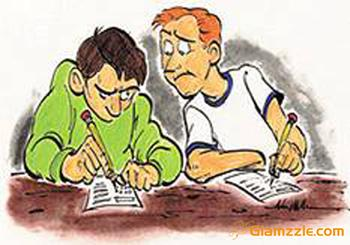# Did he know the answer?

Probability Level 3In answering a question on MCQ test with 4 choices per question, a student either knows the answer or guesses or copies the answer. Let $\cfrac { 1 }{ 2 }$ be the probability that he knows the answer, $\cfrac { 1 }{ 4 }$ be the probability that he guesses and $\cfrac { 1 }{ 4 }$ be the probability that he copies it.

Assuming that a student who copies the answer has a $\cfrac { 3 }{ 4 }$ probability to be right, what is the probability that the student knows the answer, given that he answered it correctly? Give your answer to 3 decimal places.

×

Problem Loading...

Note Loading...

Set Loading...